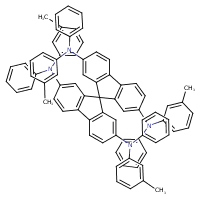Structure InformationCompound Identification

SMILES

CC1=CC(=CC=C1)N(C1=CC=CC=C1)C1=CC2=C(C=C1)C1=C(C=C(C=C1)N(C1=CC=CC=C1)C1=CC=CC(C)=C1)C21C2=C(C=CC(=C2)N(C2=CC=CC=C2)C2=CC=CC(C)=C2)C2=C1C=C(C=C2)N(C1=CC=CC=C1)C1=CC=CC(C)=C1

InChIKey

InChIKey=ZWPWBTBDUXXEIS-UHFFFAOYSA-N

Formula

C77H60N4

Mass

1041.355

### Export to:

Taxonomic Classification

Taxonomy Tree

Kingdom

Organic compounds

Superclass

Organic nitrogen compounds

Class

Organonitrogen compounds

Subclass

Amines

Intermediate Tree Nodes

Tertiary amines

Direct Parent

Triarylamines

Alternative Parents

Molecular Framework

Aromatic homopolycyclic compounds

Substituents

Tertiary aromatic amine - Fluorene - Aminotoluene - Aniline or substituted anilines - Toluene - Benzenoid - Monocyclic benzene moiety - Hydrocarbon derivative - Aromatic homopolycyclic compound

Description

This compound belongs to the class of organic compounds known as triarylamines. These are organic compounds containing a trialkylamine group, characterized by exactly three aryl groups bonded to the amino nitrogen.

External Descriptors

Not available